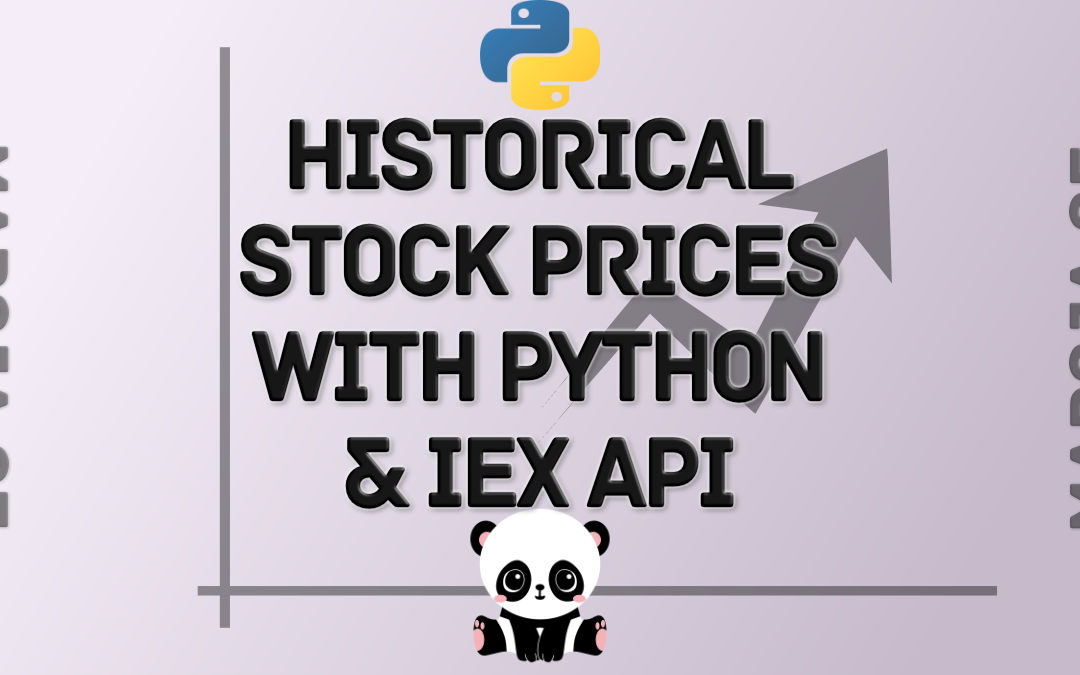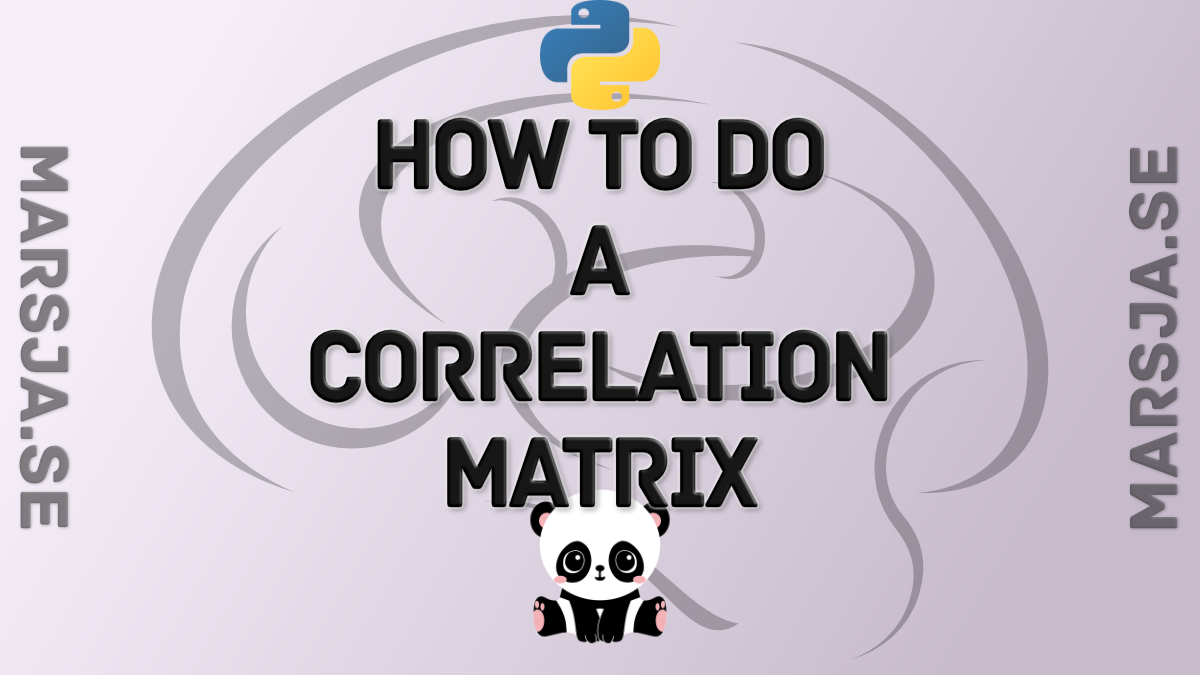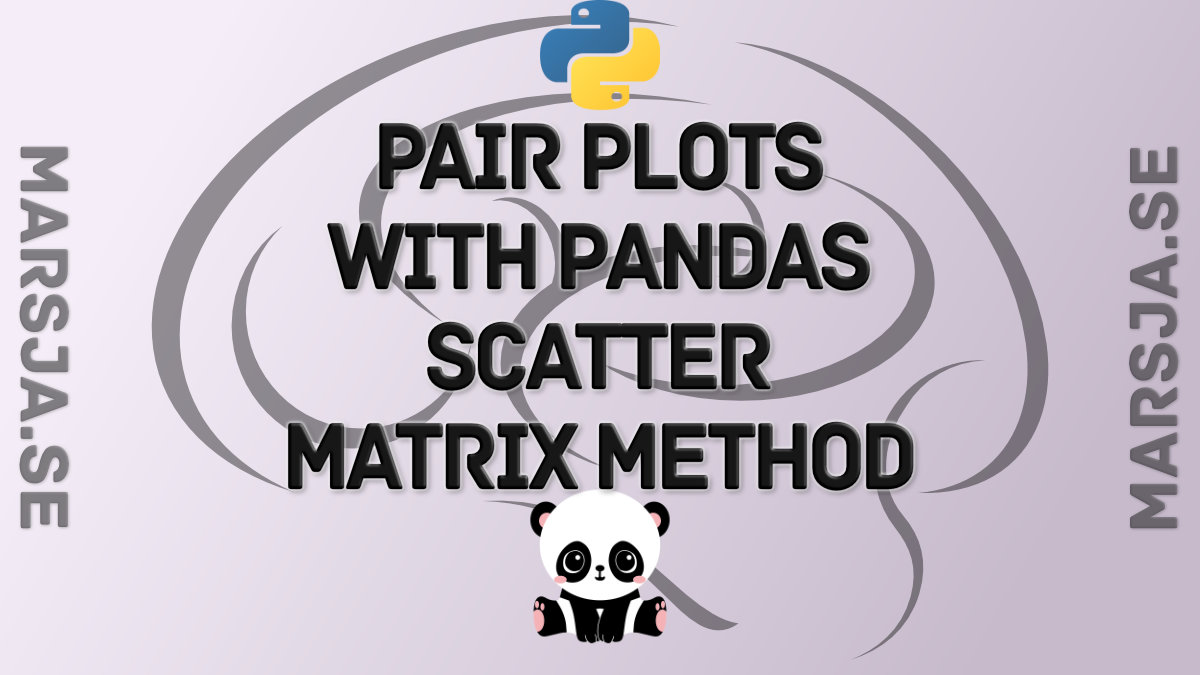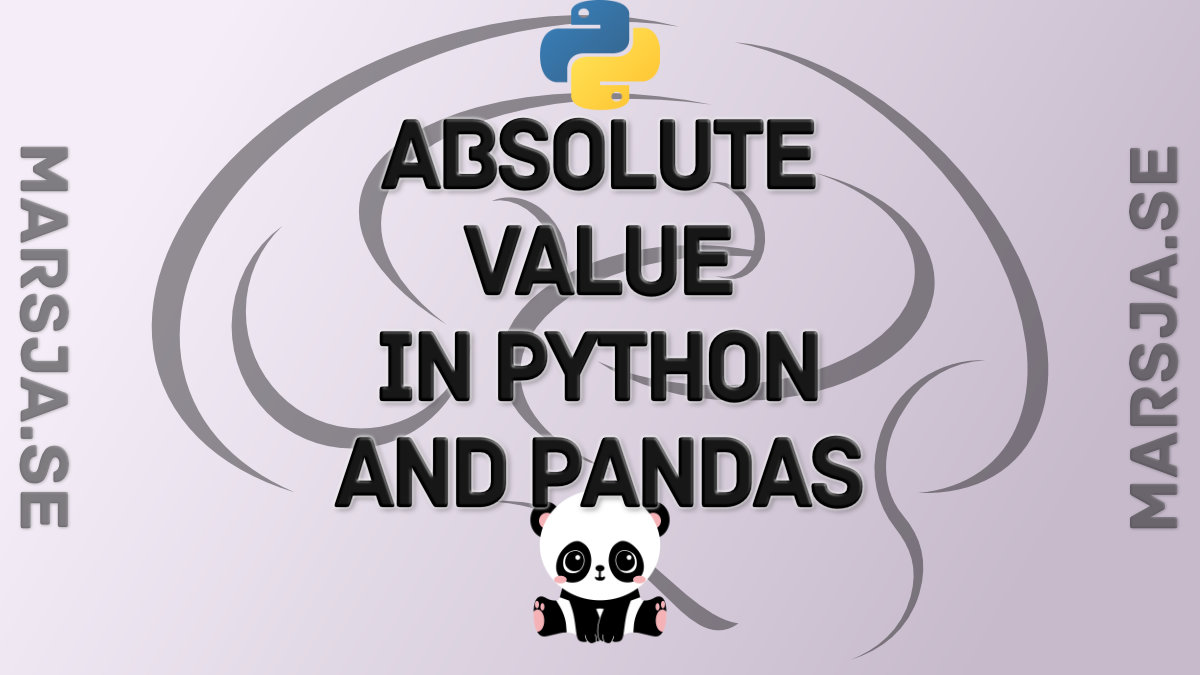## How to Import Historical Stock Prices Into A Python Script Using the IEX Cloud API

Python is one of the world’s most popular programming languages. Specifically, Python for finance is arguably the world’s most popular language-application pair. This is because of the robust ecosystem of packages and libraries that makes it easy for...## Create a Correlation Matrix in Python with NumPy and Pandas

In this post, we will go through how to calculate a correlation matrix in Python with NumPy and Pandas. Now, there will be a number of Python correlation matrix examples in this tutorial. First, we will read data from a CSV fil so we can, in a simple way, have a look...## Pandas Tutorial: Renaming Columns in Pandas Dataframe

In this Pandas tutorial, we will go through how to rename columns in a Pandas dataframe. First, we will learn how to rename a single column. Second, we will go on with renaming multiple columns. In the third example, we will also have a quick look at how to rename...## How to use Pandas Scatter Matrix (Pair Plot) to Visualize Trends in Data

In this Python data visualization tutorial, we will work with Pandas scatter_matrix method to explore trends in data. Previously, we have learned how to create scatter plots with Seaborn and histograms with Pandas, for instance. In this post, we’ll focus on scatter...## How to get Absolute Value in Python with abs() and Pandas

In this Python tutorial, we will learn how to get the absolute value in Python. First, we will use one of Pythons built-in functions abs() to do this. In this section, we will go through a couple of examples of how to get the absolute value. Second, we will import...